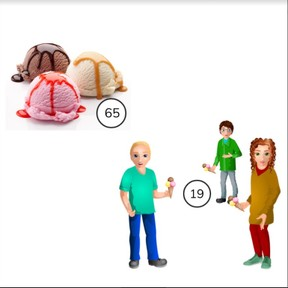Subtraction to 100 with subtrahends >10

# Subtraction to 100 with subtrahends >108,000 schools use Gynzy92,000 teachers use Gynzy1,600,000 students use Gynzy

## General

Students learn to subtract numbers to 100 with subtrahends greater than 10.

2.NBT.B.5
2.OA.A.1

## Relevance

It is important to be able to subtract numbers to 100, so you can determine how many you have left.

## Introduction

Show students the stack of 100 candies. Have them calculate how many candies are left after the first kid takes theirs away. Then subtract on from that difference (94) and take away the candies from the next student. Continue doing this until all kids have taken away some candy. How many candies are left of the 100 you started with?

## Development

Discuss the importance of subtracting numbers to 100. The learning goal will be presented in three ways, as visual, abstract and story problems. You can use the blue menu in the bottom right to select between these ways to choose the one that best suits your classroom needs.
For the visual method, start by counting all of the objects. Next explain to students how to take the second number away from the first. Show that you start by with 52 by taking away the tens. So you take away 10. Your difference is 42. Next you take away 4 more, and the difference is 38. Solve the next problem together with the students. Check that students are able to subtract using visual support by using the next subtraction problems. Next students are shown how to solve in the abstract, using a number line. Explain to students how to show subtraction as jumps on the number line. Tell students that they also jump to the previous tens number first on the number line. The next problem is abstract without a number line. Check that students are able to subtract numbers without using the number line by asking them to solve the given subtraction problems. Ask students to explain their strategy. Emphasize that there are different ways to subtract and that students should use the one that they find easiest to use. Finally in story form, explain to students what steps they should take to solve a story problem- determine what kind of math problem it is, determine which numbers are required for the math problem, to say or write the problem and then to solve the problem. Practice a story problem as a class and then have students practice individually or in pairs.

Check that students are able to solve subtraction problems to 100 with subtrahends greater than ten by asking the following questions:
- Why is it useful to be able to subtract numbers to 100?
- How do you calculate a subtraction problem with numbers to 100?

## Guided practice

Students are given subtraction problems in the visual, abstract and story problem forms. They questions start easier with multiple choice and visual support, and become more difficult with filling in the answer and abstract problems.

## Closing

Check that students have understood the learning goal by asking them to explain their strategy to subtract numbers to 100 with subtrahends greater than 10. Emphasize that every one of them might choose a different strategy, and that they should choose the method that works best for them. To close the lesson ask students to chase away the UFO by firing bombs at it to reduce their shields to less than 20. Example: Ask a student to select a bomb- they select 11. Drag the bomb to the shield and the subtraction problem is: 100 - 11 = 89. Now the shield has 89 points. Ask another student to select a bomb and continue subtracting. Depending on the numbers selected, the shield should reach below 20 after 3 or 4 bombs. At that point the UFO is defeated and flies away.

## Teaching tips

Students who have difficulty subtracting numbers to 100 can first practice subtraction problems with numbers to 50. They can also make use of MAB blocks or other manipulatives to help visualize the numbers. Help students practice crossing tens by subtracting back to the nearest tens number and then taking away the rest, or by taking away all the tens of the subtrahend and then counting back with the ones that are left.

## Instruction materials

Optional: MAB blocks or other manipulatives

### The online teaching platform for interactive whiteboards and displays in schools

• Save time building lessons

• Manage the classroom more efficiently

• Increase student engagement